# Lanson编程技术分享

## 学习目标

• 目标
• 了解偏差与方差的意义
• 知道L2正则化与L1正则化的数学意义
• 知道Droupout正则化的方法
• 了解早停止法、数据增强法的其它正则化方式
• 应用

### 2.3.1 偏差与方差

2.3.1.1 数据集划分

• 训练集（train set）：用训练集对算法或模型进行训练过程；
• 验证集（development set）：利用验证集（又称为简单交叉验证集，hold-out cross validation set）进行交叉验证选择出最好的模型
• 测试集（test set）：最后利用测试集对模型进行测试，对学习方法进行评估。

• 无验证集的情况：70% / 30%
• 有验证集的情况：60% / 20% / 20%

• 100 万数据量：98% / 1% / 1%
• 超百万数据量：99.5% / 0.25% / 0.25%

2.3.1.2 偏差与方差的意义

“偏差-方差分解”（bias-variance decomposition）是解释学习算法泛化性能的一种重要工具。

• 偏差：度量了学习算法的期望预测与真实结果的偏离程度，即刻画了学习算法本身的拟合能力
• 方差：度量了同样大小的训练集的变动所导致的学习性能的变化，即刻画了数据扰动所造成的影响
• 噪声：表达了在当前任务上任何学习算法所能够达到的期望泛化误差的下界，即刻画了学习问题本身的难度

• 1、训练集的错误率较小，而验证集/测试集的错误率较大，说明模型存在较大方差，可能出现了过拟合
• 2、训练集和测试集的错误率都较大，且两者相近，说明模型存在较大偏差，可能出现了欠拟合
• 3、训练集和测试集的错误率都较小，且两者相近，说明方差和偏差都较小，这个模型效果比较好。

2.3.1.3 解决方法

• 获取更多的数据，使得训练能够包含所有可能出现的情况
• 正则化（Regularization）
• 寻找更合适的网络结构

• 扩大网络规模，如添加隐藏层或者神经元数量
• 寻找合适的网络架构，使用更大的网络结构，如AlexNet
• 训练时间更长一些

### 2.3.2 正则化(Regularization)

2.3.2.1 逻辑回归的L1与L2正则化

• 逻辑回归的损失函数中增加L2正则化

J(w,b) = \frac{1}{m}\sum_{i=1}^mL(\hat{y}^{(i)},y^{(i)})+\frac{\lambda}{2m}{||w||}^2_2J(w,b)=​m​​1​​∑​i=1​m​​L(​y​^​​​(i)​​,y​(i)​​)+​2m​​λ​​∣∣w∣∣​2​2​​

• 逻辑回归的损失函数中增加L1正则化

J(w,b) = \frac{1}{m}\sum_{i=1}^mL(\hat{y}^{(i)},y^{(i)}) + \frac{\lambda}{2m}{||w||}_1J(w,b)=​m​​1​​∑​i=1​m​​L(​y​^​​​(i)​​,y​(i)​​)+​2m​​λ​​∣∣w∣∣​1​​

2.3.2.2 正则化项的理解

• 接下来我们通过方向传播来理解这个其中的L2，对于损失函数我们要反向传播求参数梯度：

(1) dW = \frac{\partial L}{\partial w}+ \frac{\lambda}{m} {W}dW=​∂w​​∂L​​+​m​​λ​​W

(2) W := W - \alpha dWW:=W−αdW 那么我们将第一个公式带入第二个得到

-->W := W - \alpha(\frac{\partial L}{\partial w} + \frac{\lambda}{m}W)−−>W:=W−α(​∂w​​∂L​​+​m​​λ​​W)

-->=W - \frac{\alpha \lambda}{m}W - \alpha*\frac{\partial L}{\partial w}−−>=W−​m​​αλ​​W−α∗​∂w​​∂L​​

2.3.2.3 神经网络中的正则化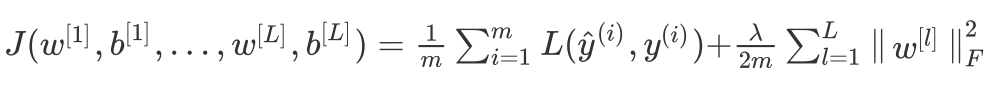{\begin{Vmatrix}w^{[l]}\end{Vmatrix}}^2_F = \sum^{n^{[l-1]}}_{i=1}\sum^{n^{[l]}}_{j=1}(w^{[l]}_{ij})^2​∥​∥​​​w​[l]​​​​​∥​∥​​​F​2​​=∑​i=1​n​[l−1]​​​​∑​j=1​n​[l]​​​​(w​ij​[l]​​)​2​​

2.3.2.4 正则化为什么能够防止过拟合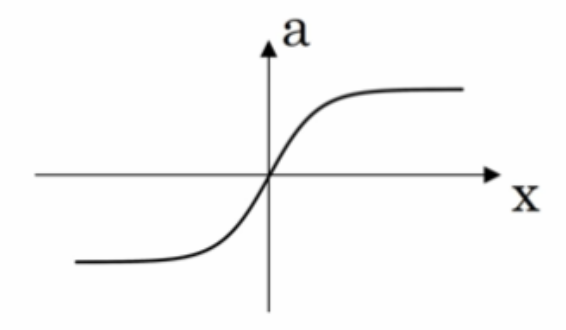### 2.3.3 Droupout正则化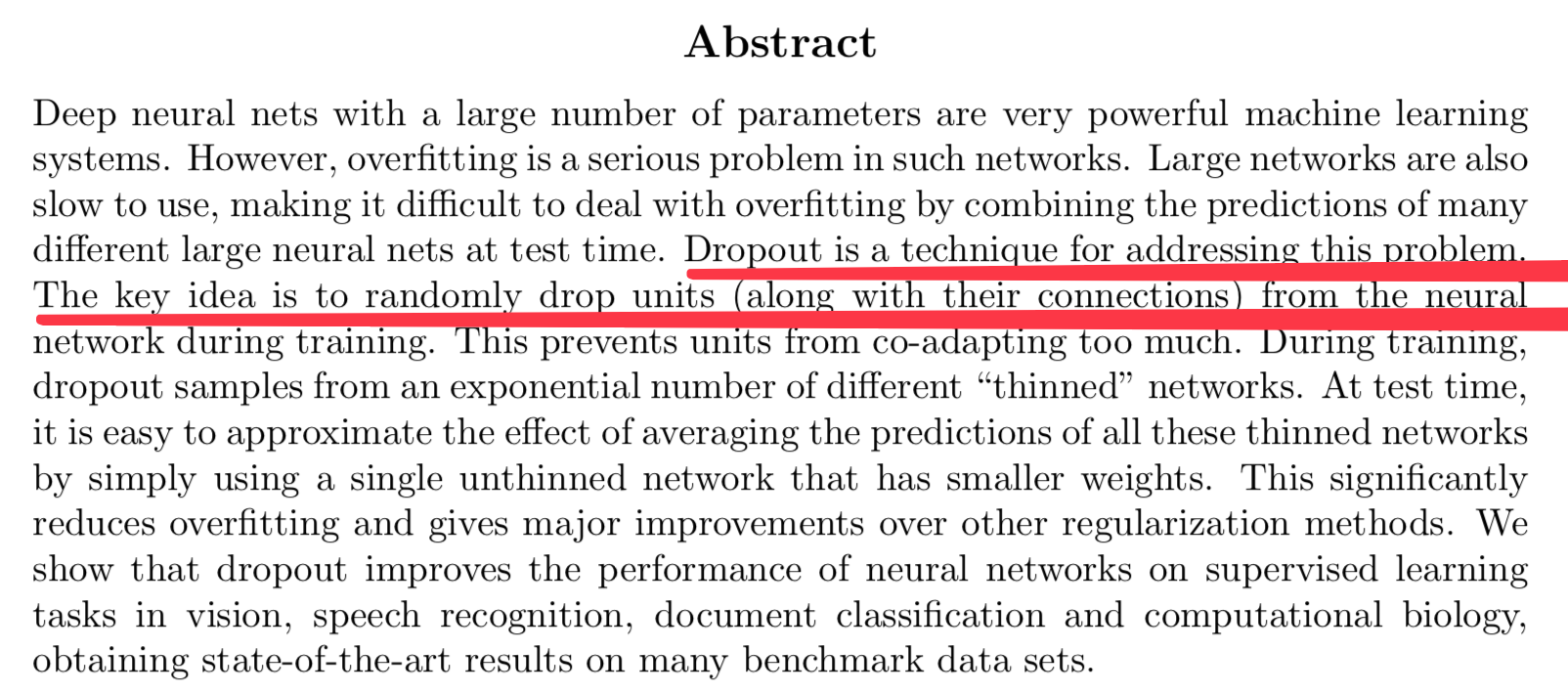Droupout：随机的对神经网络每一层进行丢弃部分神经元操作。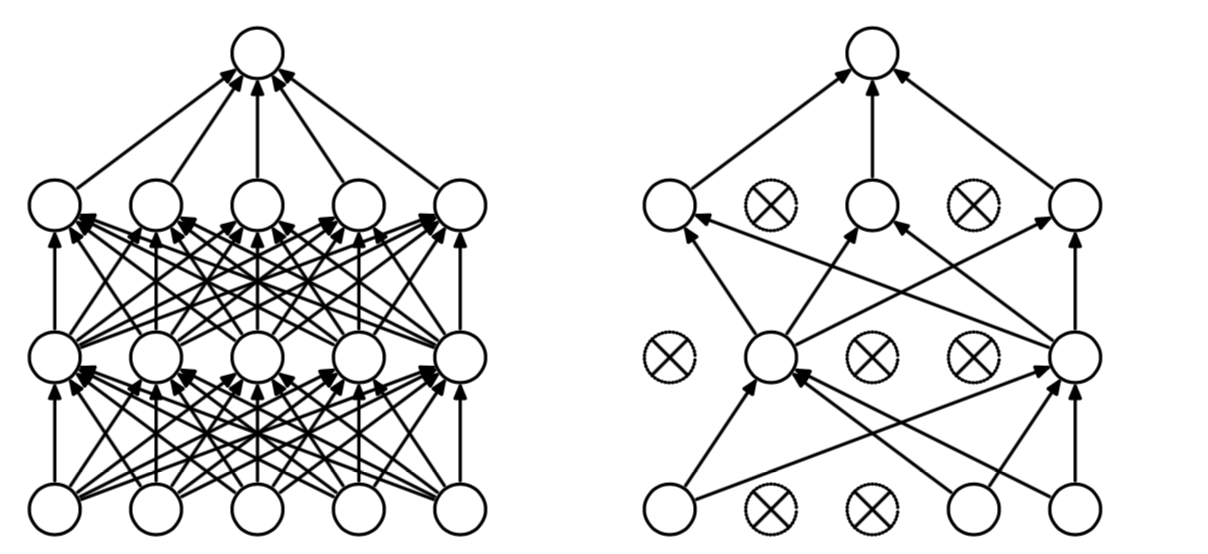2.3.3.1 Inverted droupout

# 假设设置神经元保留概率
keep_prob = 0.8
# 随机建立一个标记1 or 0的矩阵，表示随机失活的单元，占比20%
dl = np.random.rand(al.shape, al.shape) < keep_prob
# 让a1对应d1的为0地方结果为0
al = np.multiply(al, dl)

# 为了测试的时候，每一个单元都参与进来
al /= keep_prob

• 训练练的时候只有占比为pp的隐藏层单元参与训练。

• 增加最后一行代码的原因，在预测的时候，所有的隐藏层单元都需要参与进来，就需要测试的时候将输出结果除以以pp使下一层的输入规模保持不变。

z^{l}=w^{l}a^{l-1}+b^{l}z​l​​=w​l​​a​l−1​​+b​l​​ ,当$l-1$层有比例为 $1-p=0.2$单元drop后，a^{l-1}a​l−1​​大约会变为原来的80%，为了保证ll层的zz值期望（可以理解为均值）不变，所以要在a^{l-1}a​l−1​​与dropout矩阵乘积后的权重进行扩大，要乘以\frac{1}{p}=10/8​p​​1​​=10/8(增大)

2.3.3.2 droupout为什么有效总结

• 对于不同的层，设置的keep_prob大小也不一致，神经元较少的层，会设keep_prob为 1.0，而神经元多的层则会设置比较小的keep_prob

• 通常被使用在计算机视觉领域，图像拥有更多的特征，场景容易过拟合，效果被实验人员证明是很不错的。

• dropout 的缺点是成本函数无法被明确定义，因为每次会随机消除一部分神经元，所以参数也无法确定具体哪一些，在反向传播的时候带来计算上的麻烦，也就无法保证当前网络是否损失函数下降的。如果要使用droupout，会先关闭这个参数，保证损失函数是单调下降的，确定网络没有问题，再次打开droupout才会有效。

### 2.3.4 其它正则化方法

• 早停止法（Early Stopping）
• 数据增强

2.3.4.1 早停止法（Early Stopping）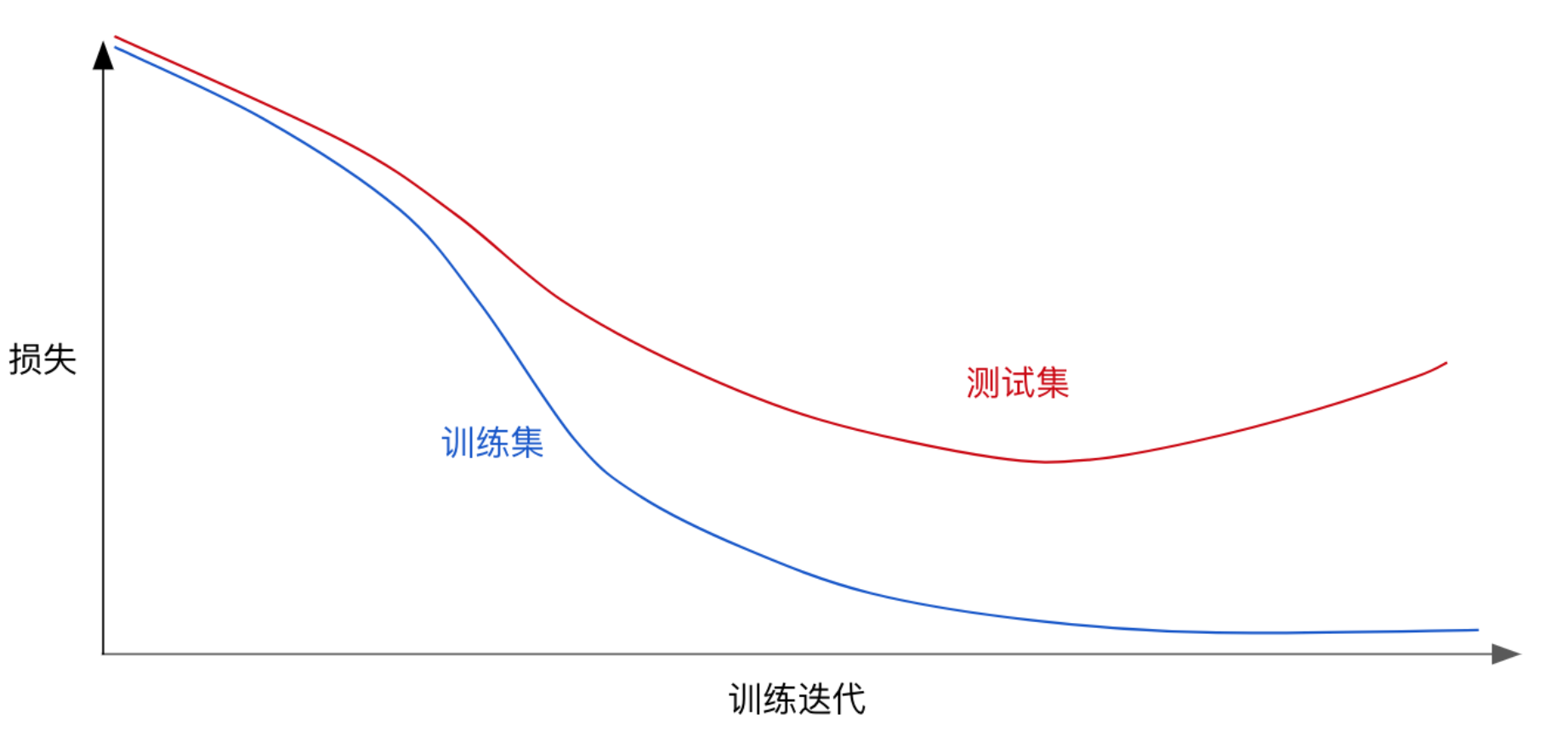2.3.4.2 数据增强

• 数据增强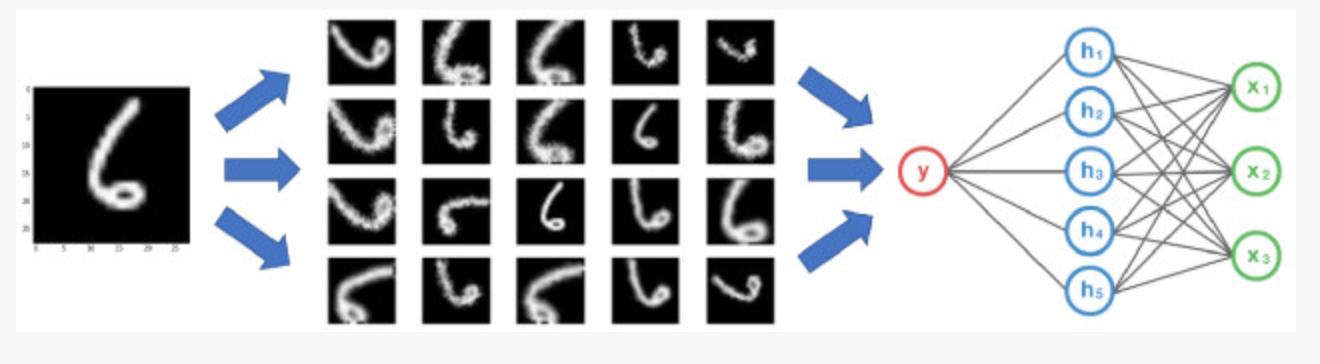• 为什么这样做？• 数据增强类别

• 离线增强。预先进行所有必要的变换，从根本上增加数据集的规模（例如，通过翻转所有图像，保存后数据集数量会增加2倍）。
• 在线增强，或称为动态增强。可通过对即将输入模型的小批量数据的执行相应的变化，这样同一张图片每次训练被随机执行一些变化操作，相当于不同的数据集了。

• 数据增强技术

• 翻转：tf.image.random_flip_left_right
• 你可以水平或垂直翻转图像。一些架构并不支持垂直翻转图像。但，垂直翻转等价于将图片旋转180再水平翻转。下面就是图像翻转的例子。从左侧开始分别是：原始图像，水平翻转图像，垂直翻转图像

• 旋转:rotat​​​​​​​

                                      从左到右，图像相对于前一个图像顺时针旋转90度

• 剪裁：random_crop
• 随机从原始图像中采样一部分，然后将这部分图像调整为原始图像大小。这个方法更流行的叫法是随机裁剪。从左侧开始分别为：原始图像，从左上角裁剪出一个正方形部分，然后从右下角裁剪出一个正方形部分。剪裁的部分被调整为原始图像大小。

• 平移、缩放等等方法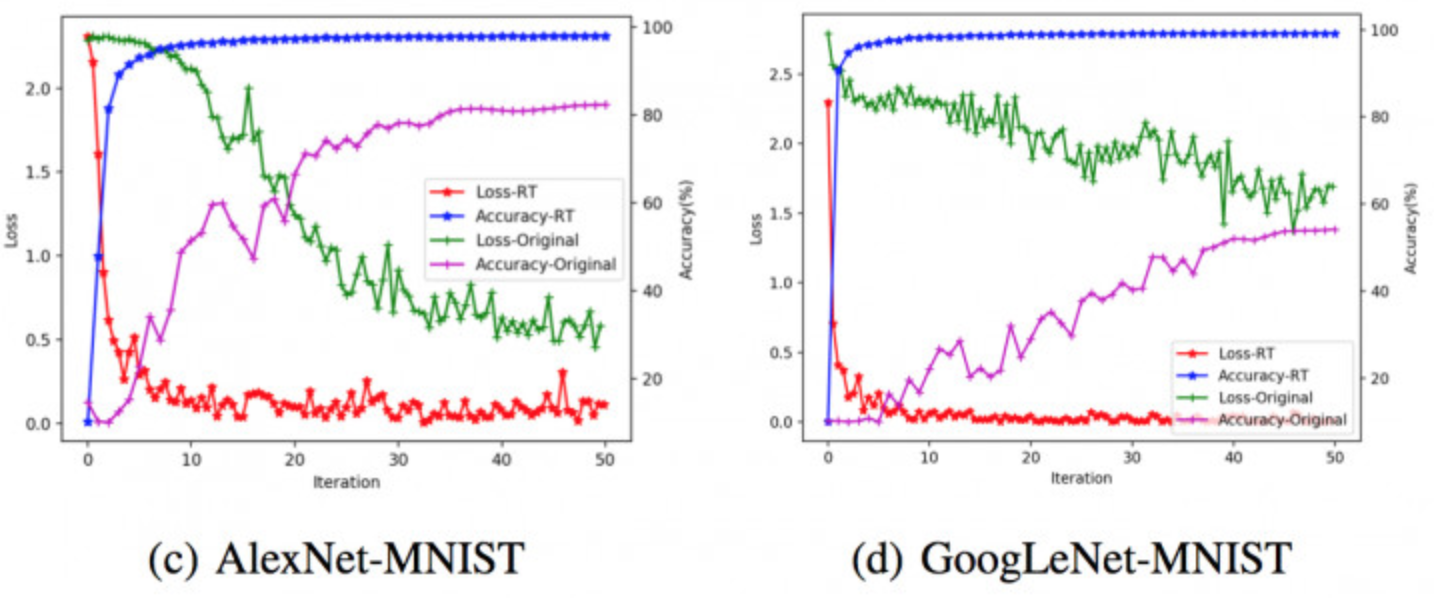### 2.3.5 总结

• 掌握偏差与方差的意义
• 掌握L2正则化与L1正则化的数学原理
• 权重衰减
• 掌握droupout原理以及方法
• Inverted droupout
• 知道正则化的作用

◎欢迎参与讨论，请在这里发表您的看法、交流您的观点。

«   2020年5月   »
123
45678910
11121314151617
18192021222324
25262728293031
文章归档
友情链接
最近发表
标签列表